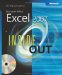# Understanding Information FunctionsThe information functions could be considered the internal monitoring system in Excel. Although they perform no specific calculations, you can use them to find out about elements of the Excel interface and then use that information elsewhere. We'll discuss the most useful of these functions in the following sections. You'll find these functions by clicking the More Functions button on the Formulas tab on the Ribbon and then clicking Information.

### Using Selected Information Functions

With information functions, you can gather information about the contents of cells, their formatting, and the computing environment as well as perform conditional tests for the presence of specific types of values.

#### The TYPE and ERROR.TYPE Functions

The TYPE function determines whether a cell contains text, a number, a logical value, an array, or an error value. The result is a code for the type of entry in the referenced cell: 1 for a number (or a blank cell), 2 for text, 4 for a logical value (TRUE or FALSE), 16 for an error value, and 64 for an array. For example, if cell A1 contains the number 100, the formula =TYPE(A1) returns 1. If A1 contains the text Microsoft Excel, the formula returns 2.

Like the TYPE function, the ERROR.TYPE function detects the contents of a cell, except it detects different types of error values. The result is a code for the type of error value in the referenced cell: 1 for #NULL!, 2 for #DIV/0!, 3 for #VALUE!, 4 for #REF!, 5 for #NAME!, 6 for #NUM!, and 7 for #N/A. Any other value in the referenced cell returns the error value #N/A. For example, if cell A1 contains a formula that displays the error value #NAME!, the formula =ERROR.TYPE(A1) returns 5. If A1 contains the text Microsoft Excel, the formula returns #N/A.

#### The COUNTBLANK Function

The COUNTBLANK function counts the number of empty cells in the specified range, which is its only argument. This function is tricky because formulas that evaluate to null text strings, such as =" ", or to zero might seem empty, but they aren't and therefore won't be counted.

### Using the IS Information Functions

You can use the ISBLANK, ISERR, ISERROR, ISEVEN, ISEOGICAL, ISNA, ISNONTEXT, ISNUMBER, ISODD, ISREF, and ISTEXT functions to determine whether a referenced cell or range contains the corresponding type of value.

All the IS information functions take a single argument. For example, the ISBLANK function takes the form =ISBLANK(value). The value argument is a reference to a cell. If value refers to a blank cell, the function returns the logical value TRUE; otherwise, it returns FALSE.

 TROUBLESHOOTING My IS function returns unexpected results. Although you can use a cell range (rather than a single cell) as the argument to any IS function, the result might not be what you expect. For example, you might think the ISBLANK function would return TRUE if the referenced range is empty or FALSE if the range contains any values. Instead, its behavior depends on where the range is in relation to the cell containing the formula. If the argument refers to a range that intersects the row or column containing the formula, ISBLANK uses implicit intersection to arrive at the result. In other words, the function looks at only one cell in the referenced range and only if it happens to be in the same row or column as the cell containing the function. The function ignores the rest of the range. If the range shares neither a row nor a column with the formula, however, the result is always FALSE. For more about intersection, see "Getting Explicit About Intersections" on page 452.An ISERR Example

You can use ISERR to avoid getting error values as formula results. For example, suppose you want to call attention to cells containing a particular character string, such as 12A, resulting in the word Yes appearing in the cell containing the formula. If the string isn't found, you want the cell to remain empty. You can use the IF and FIND functions to perform this task, but if the value isn't found, you get a #VALUE! error rather than a blank cell.

To solve this problem, add an ISERR function to the formula. The FIND function returns the position at which a substring is found within a larger string. If the substring isn't there, FIND returns #VALUE!. The solution is to add an ISERR function, such as =IF(ISERR(FIND("12A", A1)), " ", "Yes"). Because you're not interested in the error, which is simply a by-product of the calculation, this traps the error, leaving only the results in which you are interested.Note When you type numeric values as text, such as ="21", the IS function, unlike other functions, does not recognize them as numbers. Therefore, the formula =ISNUMBER("21") returns FALSE.MicrosoftВ® Office ExcelВ® 2007 Inside Out (Inside Out (Microsoft))
ISBN: 073562321X
EAN: 2147483647
Year: 2004
Pages: 260

Similar book on Amazon